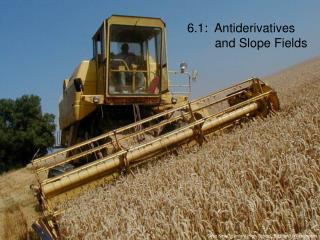Download Presentation6.1: Antiderivatives and Slope Fields

# 6.1: Antiderivatives and Slope Fields - PowerPoint PPT PresentationDownload Presentation## 6.1: Antiderivatives and Slope Fields

- - - - - - - - - - - - - - - - - - - - - - - - - - - E N D - - - - - - - - - - - - - - - - - - - - - - - - - - -
##### Presentation Transcript

1. 6.1: Antiderivatives and Slope Fields Greg Kelly, Hanford High School, Richland, Washington

2. Consider: or then: Given: find First, a little review: It doesn’t matter whether the constant was 3 or -5, since when we take the derivative the constant disappears. However, when we try to reverse the operation: We don’t know what the constant is, so we put “C” in the answer to remind us that there might have been a constant.

3. Given: and when , find the equation for . This is called an initial value problem. We need the initial values to find the constant. An equation containing a derivative is called a differential equation. It becomes an initial value problem when you are given the initial condition and asked to find the original equation. If we have some more information we can find C.

4. Slope fields are mostly used as a learning tool and are mostly done on a computer or graphing calculator, but a recent AP test asked students to draw a simple one by hand. Initial value problems and differential equations can be illustrated with a slope field.

5. Draw a segment with slope of 2. Draw a segment with slope of 0. Draw a segment with slope of 4. 0 0 0 0 1 0 0 2 0 0 3 0 2 1 0 1 1 2 2 0 4 -1 -2 0 0 -4 -2

6. If you know an initial condition, such as (1,-2), you can sketch the curve. By following the slope field, you get a rough picture of what the curve looks like. In this case, it is a parabola.

7. On the TI-89: Y= Y= Push MODE and change the Graph type to DIFF EQUATIONS. MODE Go to: and enter the equation as: Go to: Press I and make sure FIELDS is set to SLPFLD. For more challenging differential equations, we will use the calculator to draw the slope field. (Notice that we have to replace x with t , and y with y1.) (Leave yi1 blank.)

8. WINDOW GRAPH Set the viewing window: Then draw the graph:

9. Be sure to change the Graph type back to FUNCTION when you are done graphing slope fields.

10. Integrals such as are called definite integrals because we can find a definite value for the answer. The constant always cancels when finding a definite integral, so we leave it out!

11. When finding indefinite integrals, we always include the “plus C”. Integrals such as are called indefinite integrals because we can not find a definite value for the answer.

12. or Many of the integral formulas are listed on page 307. The first ones that we will be using are just the derivative formulas in reverse. On page 308, the book shows a technique to graph the integral of a function using the numerical integration function of the calculator (NINT). This is extremely slow and usually not worth the trouble. A better way is to use the calculator to find the indefinite integral and plot the resulting expression.

13. Y= COPY Use and to put this expression in the screen, and then plot the graph. PASTE To find the indefinite integral on the TI-89, use: The calculator will return: Notice that it leaves out the “+C”.